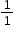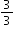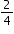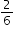# MA.3.FR.2.2

Identify equivalent fractions and explain why they are equivalent.

### Examples

Example: The fractionsandcan be identified as equivalent using number lines.

Example: The fractionsandcan be identified as not equivalent using a visual model.

### Clarifications

Clarification 1: Instruction includes identifying equivalent fractions and explaining why they are equivalent using manipulatives, drawings, and number lines.

Clarification 2: Within this benchmark, the expectation is not to generate equivalent fractions.

Clarification 3: Fractions are limited to fractions less than or equal to one with denominators of 2, 3, 4, 5, 6, 8, 10 and 12. Number lines must be given and scaled appropriately.

General Information
Subject Area: Mathematics (B.E.S.T.)
Strand: Fractions
Status: State Board Approved

## Related Courses

This benchmark is part of these courses.
5012050: Grade Three Mathematics (Specifically in versions: 2014 - 2015, 2015 - 2022, 2022 and beyond (current))
7712040: Access Mathematics Grade 3 (Specifically in versions: 2014 - 2015, 2015 - 2018, 2018 - 2022, 2022 and beyond (current))
5012055: Grade 3 Accelerated Mathematics (Specifically in versions: 2019 - 2022, 2022 and beyond (current))
5012015: Foundational Skills in Mathematics 3-5 (Specifically in versions: 2019 - 2022, 2022 and beyond (current))

## Related Access Points

Alternate version of this benchmark for students with significant cognitive disabilities.
MA.3.FR.2.AP.2: Using a visual model, recognize fractions less than a whole that are equivalent to fractions with denominators of 2, 3 or 4 (e.g., 4/8 is equivalent to 1/2).

## Related Resources

Vetted resources educators can use to teach the concepts and skills in this benchmark.

## Formative Assessments

Four Fourths:

Students are given a number line scaled by fourths and ask to label the notches.

Type: Formative Assessment

Generating Equivalent Fractions:

Students are given a familiar fraction and asked to generate an equivalent fraction justifying their reasoning.

Type: Formative Assessment

Equivalent Fractions:

Students determine whether or not fractions are equivalent.

Type: Formative Assessment

## Image/Photograph

Clipart ETC Fractions:

Illustrations that can be used for teaching and demonstrating fractions. Fractional representations are modeled in wedges of circles ("pieces of pie") and parts of polygons. There are also clipart images of numerical fractions, both proper and improper, from halves to twelfths. Fraction charts and fraction strips found in this collection can be used as manipulatives and are ready to print for classroom use.

Type: Image/Photograph

## Lesson Plan

Fraction Action!:

This lesson will help students understand that fractions are parts of a whole. The lesson introduces fractional parts using familiar manipulatives.

Type: Lesson Plan

## Original Student Tutorials

Lesson 22 Video: MEA Animal Meal Planning Part 2:

In this video, SaM-1 introduces a part 2 twist to the Model Eliciting Activity (MEA). In the optional twist, students will need to modify their original diet for a senior chimpanzee. The first video provided meal planning information to add to the knowledge students gained throughout the unit to start the challenge.

Type: Original Student Tutorial

Lesson 22 Video: MEA Animal Meal Planning:

In this video, SaM-1 introduces a Model Eliciting Activity (MEA) challenge for the students. This video provides meal planning information to add to the knowledge students gained throughout the unit. Students will be asked to develop a varied diet for a chimpanzee at the CPALMS Rehabilitation and Conservation Center based on the color, shape, texture, and hardness of the food.

In the optional twist, students will need to modify their original diet for a senior chimpanzee. The optional twist also has a SaM-1 video to introduce the twist challenge.

Type: Original Student Tutorial

Equal Fractions in Disguise:

Learn how different-sized fractional parts can represent the same amount of a whole, different-sized fractional parts in different orientations can represent the same amount of a whole, and a number line can be used to represent fractional parts of a whole in this interactive tutorial.

Type: Original Student Tutorial

## Virtual Manipulative

Fraction Game:

This virtual manipulative allows individual students to work with fraction relationships. (There is also a link to a two-player version.)

Type: Virtual Manipulative

## MFAS Formative Assessments

Equivalent Fractions:

Students determine whether or not fractions are equivalent.

Four Fourths:

Students are given a number line scaled by fourths and ask to label the notches.

Generating Equivalent Fractions:

Students are given a familiar fraction and asked to generate an equivalent fraction justifying their reasoning.

## Original Student Tutorials Mathematics - Grades K-5

Equal Fractions in Disguise:

Learn how different-sized fractional parts can represent the same amount of a whole, different-sized fractional parts in different orientations can represent the same amount of a whole, and a number line can be used to represent fractional parts of a whole in this interactive tutorial.

## Student Resources

Vetted resources students can use to learn the concepts and skills in this benchmark.

## Original Student Tutorials

Lesson 22 Video: MEA Animal Meal Planning Part 2:

In this video, SaM-1 introduces a part 2 twist to the Model Eliciting Activity (MEA). In the optional twist, students will need to modify their original diet for a senior chimpanzee. The first video provided meal planning information to add to the knowledge students gained throughout the unit to start the challenge.

Type: Original Student Tutorial

Lesson 22 Video: MEA Animal Meal Planning:

In this video, SaM-1 introduces a Model Eliciting Activity (MEA) challenge for the students. This video provides meal planning information to add to the knowledge students gained throughout the unit. Students will be asked to develop a varied diet for a chimpanzee at the CPALMS Rehabilitation and Conservation Center based on the color, shape, texture, and hardness of the food.

In the optional twist, students will need to modify their original diet for a senior chimpanzee. The optional twist also has a SaM-1 video to introduce the twist challenge.

Type: Original Student Tutorial

Equal Fractions in Disguise:

Learn how different-sized fractional parts can represent the same amount of a whole, different-sized fractional parts in different orientations can represent the same amount of a whole, and a number line can be used to represent fractional parts of a whole in this interactive tutorial.

Type: Original Student Tutorial

## Virtual Manipulative

Fraction Game:

This virtual manipulative allows individual students to work with fraction relationships. (There is also a link to a two-player version.)

Type: Virtual Manipulative

## Parent Resources

Vetted resources caregivers can use to help students learn the concepts and skills in this benchmark.

## Image/Photograph

Clipart ETC Fractions:

Illustrations that can be used for teaching and demonstrating fractions. Fractional representations are modeled in wedges of circles ("pieces of pie") and parts of polygons. There are also clipart images of numerical fractions, both proper and improper, from halves to twelfths. Fraction charts and fraction strips found in this collection can be used as manipulatives and are ready to print for classroom use.

Type: Image/Photograph